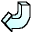Back# Solving Linear Equations

A linear equation looks like this:
x + 3 = 9

or this:
2x - 7 = 11

or even this:
4x - 1 = x +13

When we solve one of these equations, we have to find out what number we can replace the x with, so that both sides of the equation give us the same answer.

There will only be one number that will do this. We could try to find it by trial and error, by seeing what happens when we replace x by different numbers until we find one that matches both sides. But that could take us a long time.

A better way is to perform what mathematicians call inverse operations. In other words, whatever has been done to x, you must do the opposite!

So, if 3 has been added to x, you must subtract 3 from both sides of the equation. If 7 has been taken away from x, you must add 7 to both sides. If x has been multiplied by 2, you must divide the whole equation by 2.

An equation is like a balance. Whatever you do to one side, you must remember to do exactly the same to the other side, or the whole thing goes wrong!

Try these examples, and click on the answer button for each to see if you got them right.

3x + 2 = 113x - 2 = x + 10